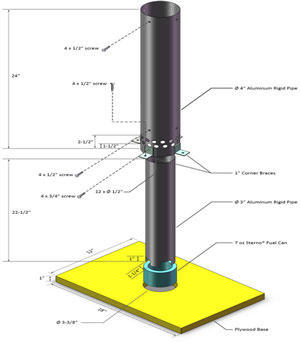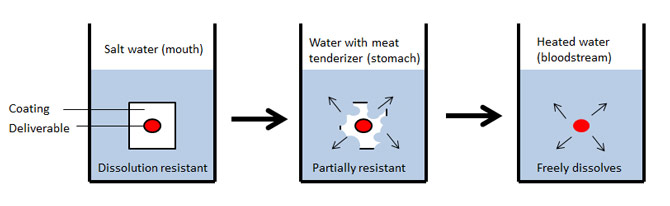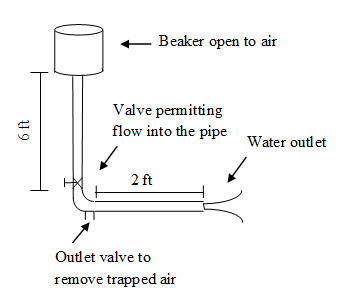STEM Modules

The following STEM modules are currently in development. All will be free to use but may have some initial cost in terms of supplies – all of which you should be able to obtain in your region:

SUPERBALLS: (cost = ~\$10)Subject matter: area, mass, synthesis, concentration, elasticity
Fields of interest: math, physics, chemistry, engineering

HOT AIR BALLOONS: (cost = ~\$20)
Subject matter: ideal gas law, density, temperature
Fields of interest: physics, engineering

DRUG DELIVERY: (cost = ~\$10)
Subject matter: medicine, human physiology and anatomy
Fields of interest: biology, chemistry, engineeringDRAG REDUCING POLYMERS: (cost = ~\$10)Subject matter: friction, fluid
Fields of interest: physics, chemistry, engineering

RENEWABLE FERTILIZER: (cost = ~\$30)
Subject matter: renewable energy, biology
Fields of interest: plant science, environmental science, chemistry